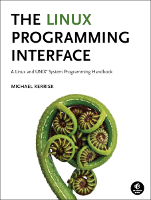# svsem/svsem_op.c

This is svsem/svsem_op.c (Listing 47-8, page 982), an example from the book, The Linux Programming Interface.

This page shows the "distribution" or "book" version of the file (why are there two versions?), or the differences between the two versions. You can switch between the views using the tabs below.

In the listing below, the names of Linux system calls and C library functions are hyperlinked to manual pages from the Linux man-pages project, and the names of functions implemented in the book are hyperlinked to the implementations of those functions.## Function list (Bold in this list means a function is not static)

```/* svsem_op.c

Perform groups of operations on a System V semaphore set.

Usage as shown in usageError().
*/
#include <sys/types.h>
#include <sys/sem.h>
#include <ctype.h>
#include "curr_time.h"          /* Declaration of currTime() */
#include "tlpi_hdr.h"

#define MAX_SEMOPS 1000         /* Maximum operations that we permit for
a single semop() */
```
```static void
usageError(const char *progName)
{
fprintf(stderr, "Usage: %s semid op[,op...] ...\n\n", progName);
fprintf(stderr, "'op' is either: <sem#>{+|-}<value>[n][u]\n");
fprintf(stderr, "            or: <sem#>=0[n]\n");
fprintf(stderr, "       \"n\" means include IPC_NOWAIT in 'op'\n");
fprintf(stderr, "       \"u\" means include SEM_UNDO in 'op'\n\n");
fprintf(stderr, "The operations in each argument are "
"performed in a single semop() call\n\n");
fprintf(stderr, "e.g.: %s 12345 0+1,1-2un\n", progName);
fprintf(stderr, "      %s 12345 0=0n 1+1,2-1u 1=0\n", progName);
exit(EXIT_FAILURE);
}
```
```/* Parse comma-delimited operations in 'arg', returning them in the
array 'sops'. Return number of operations as function result. */

static int
parseOps(char *arg, struct sembuf sops[])
{
char *comma, *sign, *remaining, *flags;
int numOps;                 /* Number of operations in 'arg' */

for (numOps = 0, remaining = arg; ; numOps++) {
if (numOps >= MAX_SEMOPS)
cmdLineErr("Too many operations (maximum=%d): \"%s\"\n",
MAX_SEMOPS, arg);

if (*remaining == '\0')
fatal("Trailing comma or empty argument: \"%s\"", arg);
if (!isdigit((unsigned char) *remaining))
cmdLineErr("Expected initial digit: \"%s\"\n", arg);

sops[numOps].sem_num = strtol(remaining, &sign, 10);

if (*sign == '\0' || strchr("+-=", *sign) == NULL)
cmdLineErr("Expected '+', '-', or '=' in \"%s\"\n", arg);
if (!isdigit((unsigned char) *(sign + 1)))
cmdLineErr("Expected digit after '%c' in \"%s\"\n", *sign, arg);

sops[numOps].sem_op = strtol(sign + 1, &flags, 10);

if (*sign == '-')                       /* Reverse sign of operation */
sops[numOps].sem_op = - sops[numOps].sem_op;
else if (*sign == '=')                  /* Should be '=0' */
if (sops[numOps].sem_op != 0)
cmdLineErr("Expected \"=0\" in \"%s\"\n", arg);

sops[numOps].sem_flg = 0;
for (;; flags++) {
if (*flags == 'n')
sops[numOps].sem_flg |= IPC_NOWAIT;
else if (*flags == 'u')
sops[numOps].sem_flg |= SEM_UNDO;
else
break;
}

if (*flags != ',' && *flags != '\0')
cmdLineErr("Bad trailing character (%c) in \"%s\"\n", *flags, arg);

comma = strchr(remaining, ',');
if (comma == NULL)
break;                              /* No comma --> no more ops */
else
remaining = comma + 1;
}

return numOps + 1;
}
```
```int
main(int argc, char *argv[])
{
struct sembuf sops[MAX_SEMOPS];
int ind, nsops;

if (argc < 2 || strcmp(argv, "--help") == 0)
usageError(argv);

for (ind = 2; argv[ind] != NULL; ind++) {
nsops = parseOps(argv[ind], sops);

printf("%5ld, %s: about to semop()  [%s]\n", (long) getpid(),
currTime("%T"), argv[ind]);

if (semop(getInt(argv, 0, "semid"), sops, nsops) == -1)
errExit("semop (PID=%ld)", (long) getpid());

printf("%5ld, %s: semop() completed [%s]\n", (long) getpid(),
currTime("%T"), argv[ind]);
}

exit(EXIT_SUCCESS);
}```

Note that, in most cases, the programs rendered in these web pages are not free standing: you'll typically also need a few other source files (mostly in the lib/ subdirectory) as well. Generally, it's easier to just download the entire source tarball and build the programs with make(1). By hovering your mouse over the various hyperlinked include files and function calls above, you can see which other source files this file depends on.